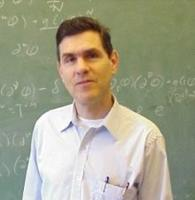# 2010 Peterson Public Lecture

Dr. Carl Bender

Physics Department, Washington University in St. LouisIn the Complex World Classical Mechanics and Quantum Mechanics are Very Much Alike!

Tuesday, April 27, 2010

3:00 p.m.

Hale Library - Hemisphere Room

Classical mechanics and quantum mechanics are two very different theories: In classical mechanics the motion of a particle is governed by Newton's laws and we can say exactly where the particle is and how fast it is going at all times. The energy of a classical particle in a potential takes on a continuum of values. In quantum mechanics, on the other hand, particles display wavelike properties and predictions are probabilistic in character. The energy of a quantum particle in a potential can only take on a discrete set of allowed "quantized" values.

Many years ago, mathematicians generalized the real number system to the complex number system. By doing this, mathematicians can understand and explain the real number system more clearly.

What happens if we follow the mathematicians and generalize real physics to complex physics? In this talk I will show what happens when we generalize ordinary real classical mechanics to complex classical mechanics. We will see that, despite the enormous differences between classical mechanics and quantum mechanics in the real world, these differences melt away in the complex world and these two theories behave in an eerily similar fashion.

This talk will be presented at an elementary popular science level.

Lecture Poster